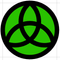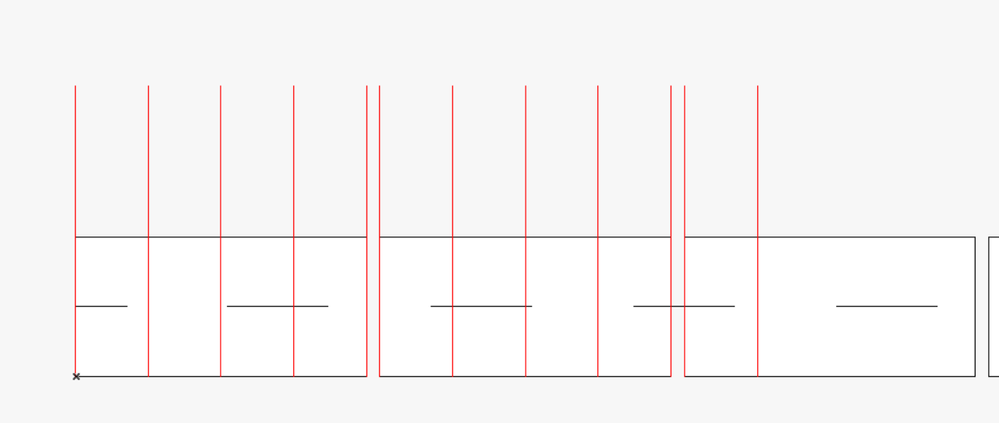Developer forum
cancel
Showing results for
Did you mean:

"Locking" available dimensions to set pointsHello,

I am trying to lock a point to only be set to specific points. Usually I would just use Step in a Values line to define it but the points aren't evenly spaced throughout it all.

Here is the script I currently have but I'm having some issues with it and I don't know where I'm going wrong:

(brick_position holds each possible dimension that the opening_point_2 variable should be able to be set to.

I would adjust the opening_point_2 variable using a hotspot in 2D plan. )

base_point = 0
j=1
DIM brick_position[]
for i=1 to num_bricks
brick_position[j] = base_point	! start of brick
j = j+1
base_point = base_point + (brick_w*0.25)
brick_position[j] = base_point ! quarter of brick
j=j+1
base_point = base_point + (brick_w*0.25)
brick_position[j] = base_point ! half of brick
j=j+1
base_point = base_point + (brick_w*0.25)
brick_position[j] = base_point ! three quarters of brick
j=j+1
base_point = base_point + (brick_w*0.25)
brick_position[j] = base_point ! Full brick
j=j+1
next i
parameters brick_position=brick_position

if (glob_modpar_name = "opening_point_2") then
k=1
repeat
k=k+1
until ((opening_point_2 >= brick_position[k]) and (opening_point_2 < brick_position[k+1])) or (k > vardim1(brick_position))

opening_point_2 = brick_position[k]

parameters counter=counter
parameters opening_point_2=opening_point_2
parameters k=k
endif

ArchiCAD 17, 19, 21 and 23
Windows 8
1 ACCEPTED SOLUTION
SolutionVirtuoso

@JGoode Sorted & tested. I had missed a pair of brackets in the dQtrBricks calculation. Revised code attached taken from my Master Script.

!Declared Variables
! dBrickTotal  : Set in Parameters with optional dynamic hotspot in 2D script

_dJoint = 0.01		!Mortar joint width
_dBrickLen = 0.300	!Full brick length without mortar

!Calculate length of uncut bricks
_dIntBricks = int(dBrickTotal / (_dBrickLen + _dJoint)) * (_dBrickLen + _dJoint)

!Calculate remaining brick length
_dFraBricks = dBrickTotal - _dIntBricks - _dJoint

!Round cut brick to quarter brick length
_dQtrBricks = round_int(_dFraBricks / (_dBrickLen * 0.25)) * _dBrickLen * 0.25

!Set length to full bricks + mortar + cut brick
if _dQtrBricks < _dBrickLen * 0.25 then
if dBrickTotal < _dIntBricks then
dBrickTotal = _dIntBricks - _dJoint
else
dBrickTotal = _dIntBricks
endif
else
dBrickTotal = _dIntBricks + _dQtrBricks
endif

parameters dBrickTotal = dBrickTotal

Apple iMac macOS Big Sur / AC24UKI (most recent builds)
7 REPLIES 7Virtuoso

I would be more inclined to set "opening_point_2" in the parameter script with maths rather than using a loop e.g.

opening_point_2 = int(opening_point_2 / brick_w * 0.25) * opening_point_2
parameters opening_point_2 = opening_point_2

Obviously you will need to fine tune using the parameter with the base offsets, but the value should round to the nearest quarter brick when stretching or manually entered.

You could consider ROUND_INT as an alternative.

Apple iMac macOS Big Sur / AC24UKI (most recent builds)Virtuoso

Can't seem to edit replies anymore? First line corrected...

opening_point_2 = int(opening_point_2 / brick_w * 0.25) * brick_w * 0.25
parameters opening_point_2 = opening_point_2

Apple iMac macOS Big Sur / AC24UKI (most recent builds)This works apart from the fact it doesn't take into account the mortar_joint. Therefore it doesn't quite go up in quarters... it goes 0.25, 0.25, 0.25, 0.25, mortar joint space, 0.25, 0.25, 0.25, 0.25, mortar joint space etc. Which is the main reason I'm struggling with it. Your bit of code works perfectly if I didn't need to account for the gap

ArchiCAD 17, 19, 21 and 23
Windows 8Virtuoso

I haven't tested it but see if this works...

dJoint = 0.010 !Brick joint width (preset parameter?)

!Calculate length of uncut bricks
dIntBricks = int(opening_point_2 / (brick_w + dJoint)) * (brick_w + dJoint)

!Calculate remaining brick length
dFraBricks = fra(opening_point_2 / (brick_w + dJoint)) - dJoint

!Round cut brick to quarter brick length
dQtrBricks = int(dFraBricks / brick_w * 0.25) * brick_w * 0.25

!Set length to full bricks + mortar + cut brick
if dQtrBricks < brick_w * 0.25 then
opening_point_2 = dIntBricks
else
opening_point_2 = dIntBricks + dJoint + dQtrBricks
endif

parameters opening_point_2 = opening_point_2

Apple iMac macOS Big Sur / AC24UKI (most recent builds)Thank you very much for taking the time to write this. Unfortunately it doesn't "jump" up in increments like I would like. I've attached an image, the red lines are the points that I would like the point to be locked to and then obviously to continue along the length of the object.ArchiCAD 17, 19, 21 and 23
Windows 8
SolutionVirtuoso

@JGoode Sorted & tested. I had missed a pair of brackets in the dQtrBricks calculation. Revised code attached taken from my Master Script.

!Declared Variables
! dBrickTotal  : Set in Parameters with optional dynamic hotspot in 2D script

_dJoint = 0.01		!Mortar joint width
_dBrickLen = 0.300	!Full brick length without mortar

!Calculate length of uncut bricks
_dIntBricks = int(dBrickTotal / (_dBrickLen + _dJoint)) * (_dBrickLen + _dJoint)

!Calculate remaining brick length
_dFraBricks = dBrickTotal - _dIntBricks - _dJoint

!Round cut brick to quarter brick length
_dQtrBricks = round_int(_dFraBricks / (_dBrickLen * 0.25)) * _dBrickLen * 0.25

!Set length to full bricks + mortar + cut brick
if _dQtrBricks < _dBrickLen * 0.25 then
if dBrickTotal < _dIntBricks then
dBrickTotal = _dIntBricks - _dJoint
else
dBrickTotal = _dIntBricks
endif
else
dBrickTotal = _dIntBricks + _dQtrBricks
endif

parameters dBrickTotal = dBrickTotal

Apple iMac macOS Big Sur / AC24UKI (most recent builds)This is brilliant. Thank you very much! I never would have been able to figure this out.

ArchiCAD 17, 19, 21 and 23
Windows 8Latest solutions

Most liked posts